# PSAT Math : How to multiply binomials with the distributive property

## Example Questions

### Example Question #1 : Binomials

Decrease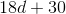by 40%. Which of the following will this be equal to?

Possible Answers: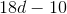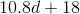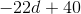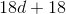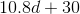Correct answer:Explanation:

A number decreased by 40% is equivalent to 100% of the number minus 40% of the number. This is taking 60% of the number, or, equivalently, multiplying it by 0.6.

Therefore,decreased by 40% is 0.6 times this, or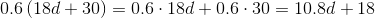### Example Question #2 : Binomials

Find the product: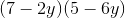Possible Answers: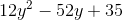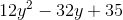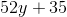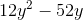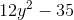Correct answer:Explanation:

Find the product:Use the distributive property: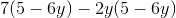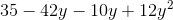Write the resulting expression in standard form:### All PSAT Math Resources